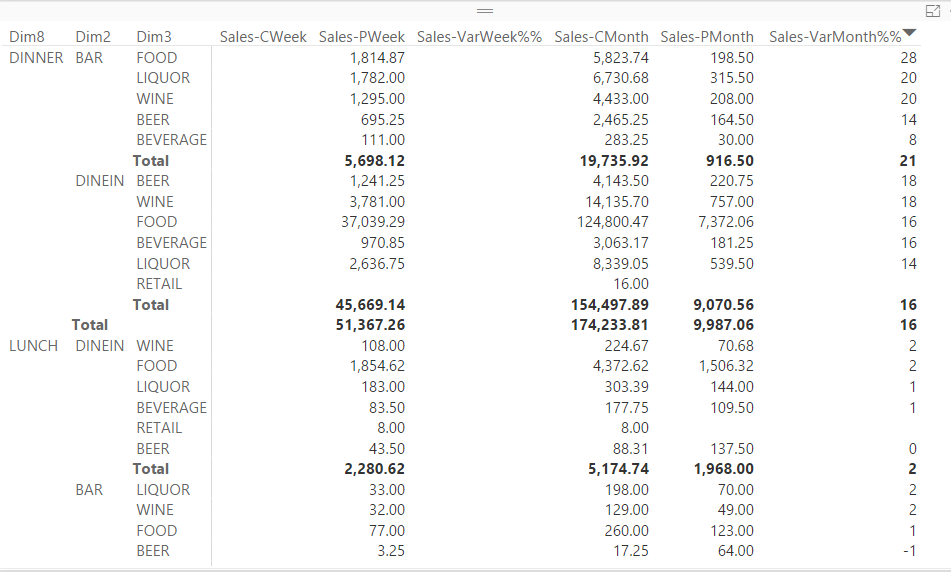cancel
Showing results for
Did you mean:Helper I

## Week to date so far

Ok first, please rest assured I have looked through what I presummed all possible answers in the forums first 😄

I am trying to create a matrix table that compares sales from the current week and previous week with the sales variance. Now here is my problem, the sales for current week will not populate unless that week is full ( as in monday - sunday).

My formula works on dummy data and if I change it on this current data to 4 weeks, 3 weeks, 2 weeks, 1 week back it works.

My question is how do I get the current cummulative total to show without it wanting to finish out the week?

`Sales-CWeek = CALCULATE([SalesTotal], SalesEntry[YearWeek] = WEEKNUM(NOW(),2) )`

This will be a daily refreshed argument for our clients so they can keep track of where they are at for the week verses the previous week.1 ACCEPTED SOLUTIONMicrosoft

Hi @kenthub,

Could you try the formula below to see if it works?```Sales-CWeek =
CALCULATE (
[SalesTotal],
FILTER (
'PeriodDate',
YEAR ( 'PeriodDate'[Date] ) = YEAR ( NOW () )
&& WEEKNUM ( 'PeriodDate'[Date], 2 ) = WEEKNUM ( NOW (), 2 )
)
)
```

Regards

2 REPLIES 2Microsoft

Hi @kenthub,

Could you try the formula below to see if it works?```Sales-CWeek =
CALCULATE (
[SalesTotal],
FILTER (
'PeriodDate',
YEAR ( 'PeriodDate'[Date] ) = YEAR ( NOW () )
&& WEEKNUM ( 'PeriodDate'[Date], 2 ) = WEEKNUM ( NOW (), 2 )
)
)
```

RegardsHelper I

Worked!  Thanks so much!

I tried something similar by setting a second filter for the current year in the Calculate function but it wasn't showing as correct. I presume the filter function simplifies that and/or makes it easier when crunching dates?